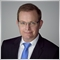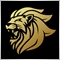# MQL4 to MQL5 EA conversion209

Hi Guys,

I'm in the process of learning how to convert my MT4 EAs into MT5 EAs.

I'm working on a simple BB EA that buys on the lower band and closes at the middle band / aka: BASE_LINE.

The EA successfully opens positions but does not close them out at the middle BB.

Can you guys please point me in the right direction - I'm at a loss!!

Thanks, Tim

```//+------------------------------------------------------------------+
//|                                                   EA Builder.mq5 |
//|                                        Copyright 2018, Tim Smith |
//|                                             https://www.mql5.com |
//+------------------------------------------------------------------+
#property version   "1.00"

input double LotSize=0.1;
input int StopLoss=30;
input int TakeProfit=10;
input int WaitTime=15;
input int MagicNumber=1234;
double poen;

#include <mq4.mqh>

int MAHandle = INVALID_HANDLE;
int MAHandle2 = INVALID_HANDLE;

//+------------------------------------------------------------------+
//| Expert initialization function                                   |
//+------------------------------------------------------------------+
int OnInit()
{

//MAHandle = iMA(NULL,0, 50, 1, MODE_SMA, PRICE_CLOSE);

MAHandle =iBands(NULL,0,20,1,2,PRICE_CLOSE);

if(MAHandle == INVALID_HANDLE)
{
Print("Error creating MA indicator");
return(INIT_FAILED);
}

MAHandle2 =iBands(NULL,0,20,1,2,PRICE_CLOSE);

if(MAHandle2 == INVALID_HANDLE)
{
Print("Error creating MA indicator");
return(INIT_FAILED);
}

if (Digits==3 || Digits==5) poen=10*Point; else poen=Point;

return(INIT_SUCCEEDED);
}
//+------------------------------------------------------------------+
//| Expert deinitialization function                                 |
//+------------------------------------------------------------------+
void OnDeinit(const int reason)
{
//---

}
//+------------------------------------------------------------------+
//| Expert tick function                                             |
//+------------------------------------------------------------------+
void OnTick()
{

CloseAtMiddleBand();

}
//+------------------------------------------------------------------+
double MAGet(const int index)
{
double ma;
// reset error code
ResetLastError();
// fill a part of the ma array with values from the indicator buffer that has 0 index
if(CopyBuffer(MAHandle,2,index,1,ma) < 0)
{
// if the copying fails, tell the error code
PrintFormat("Failed to copy data from the iMA indicator, error code %d",GetLastError());
// quit with zero result - it means that the indicator is considered as not calculated
return(0);
}
return(ma);
}

double MAGet2(const int index)
{
double bb;
// reset error code
ResetLastError();
// fill a part of the ma array with values from the indicator buffer that has 0 index
if(CopyBuffer(MAHandle,0,index,1,bb) < 0)
{
// if the copying fails, tell the error code
PrintFormat("Failed to copy data from the iMA indicator, error code %d",GetLastError());
// quit with zero result - it means that the indicator is considered as not calculated
return(0);
}
return(bb);
}

{

double tps = 0,tpb = 0,sls =0,slb =0;
slb=Bid+StopLoss*poen;
tpb=Bid-TakeProfit*poen;
static datetime TimeSent;

if(OpenOrdersThisPair(Symbol())<=3)
if (TimeCurrent() >= TimeSent + (WaitTime *60))
TimeSent = TimeCurrent();
else Print("Order ",buyticket," was NOT successfully modified.",GetLastError());

}

void CloseAtMiddleBand()

{

}

//+------------------------------------------------------------------+
//|    Close orders                                                  |
//+------------------------------------------------------------------+

{

for(int i=OrdersTotal() -1; i >= 0; i--)
{
if( OrderMagicNumber() == MagicNumber)

if (OrderClose(OrderTicket(),OrderLots(),Bid,6,CLR_NONE))
Print("Closed", OrderTicket(), "successfully");
else Print("Buy order",OrderTicket(), "was NOT closed successfully");

}
}

//+------------------------------------------------------------------+
//checks to see if any orders open on this currency pair.
//+------------------------------------------------------------------+
int OpenOrdersThisPair(string pair)
{

int total=0;

for(int i=OrdersTotal()-1; i >= 0; i--)
{
if(OrderSymbol()== pair)total++;
}
return (total);

}```Moderator
12729

You call CloseAtMiddleBand();

But CloseAtMiddleBand(); does not call the indicator.

How is it suppose to know when to close ?209

Hi Marco,

Thanks for your help on this.

Aren't I calling the indicator through "MAGet2(0)"? Can you please show me how I should call the indicator?

Thanks, Tim

```void CloseAtMiddleBand()

{

}```209

Hi MQL5 experts,

Can anyone else shed light as to why my EA is not closing at the middle BB?

Thanks, Tim